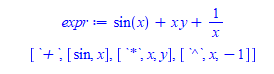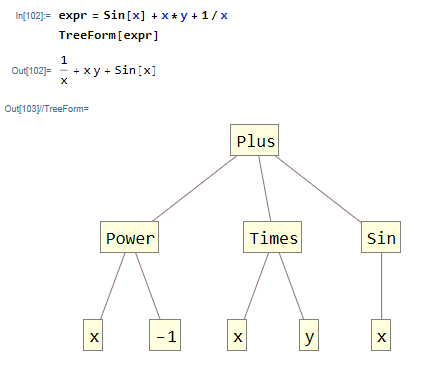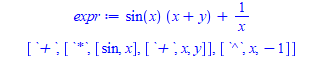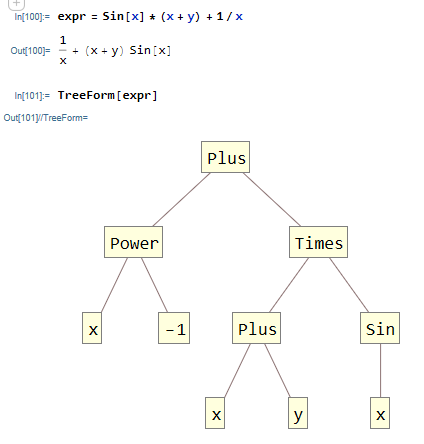# Question:converting expression parsed output to actual Graph?

## Question:converting expression parsed output to actual Graph?

Maple 2021

There is code at Maple app center  here  called "A Simple Expression Parser" which generates the actual tree structure of an expression. I tested it a little and it seems to work correctly on what I tried so far.

My question if some Graph expert could take the output of the above and generate an actual tree graph from it, to make it easier to see, similar to Mathematica TreeForm command which would make it much more useful.

I will show 2 examples, and the code from the above application and what the final graph should look like,. The code is (formatted a little to make it easier to read)

```#code from https://www.maplesoft.com/applications/view.aspx?SID=4808
Op := proc(x)
if 1 < nops(x) and not type(x, function) then
[whattype(x), op(x)];
elif type(x, function) then
[op(0, x), op(x)];
else
x;
fi;
end proc;

Parse := proc(expr)
local tmp, i;
tmp := Op(expr);
for i from 2 to nops(tmp) do
if 1 < nops(tmp[i]) or type(tmp[i], function) then
tmp := subsop(i = Parse(tmp[i]), tmp);
end if;
od;
RETURN(tmp);
end proc;
```

ps. I do not think using name Parse above is good idea, since I see it is an inert form of Maple build in command.

Now, lets look at this

first example

```expr:=sin(x)+x*y + 1/x;
Parse(expr)
```The above says the tree is rooted at `+` with three branches. The first is sin(x), the second is a tree rooted at `*` with two leafs x,y, and the third branch is roots at `^` with two leafs x,-1. Physically it looks like thisSecond example

```expr := sin(x)*(x + y) + 1/x;
Parse(expr)
```Which physically will look likeSo it is possible in theory to make a TreeForm command in Maple, using this Parse() command. May be using Graph package in Maple? by reading the output from the Parse() command, and generating nodes and arcs along the way.

How hard will such a task be? I never used the Maple 's Graph package.

Could may be  someone may be give this an attempt? I never understood why Maple do not have a build in similar command to TreeForm. It is very useful to understanding expressions.﻿